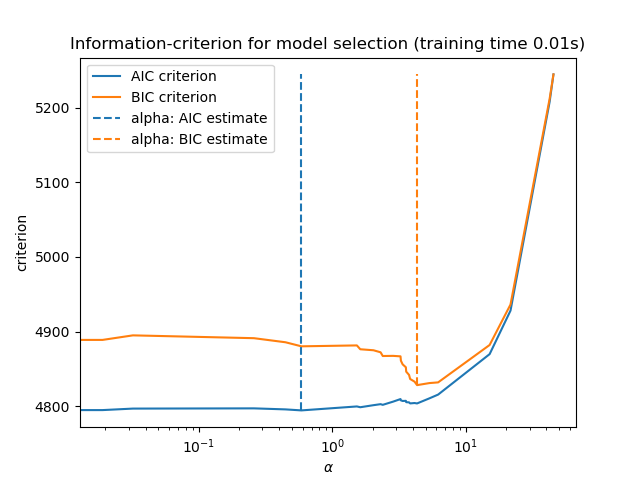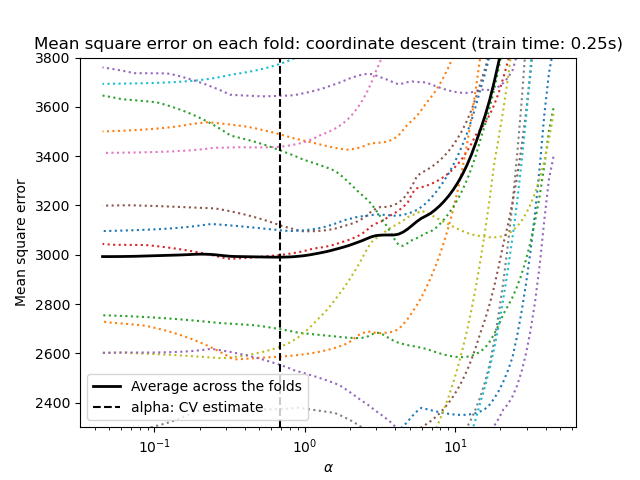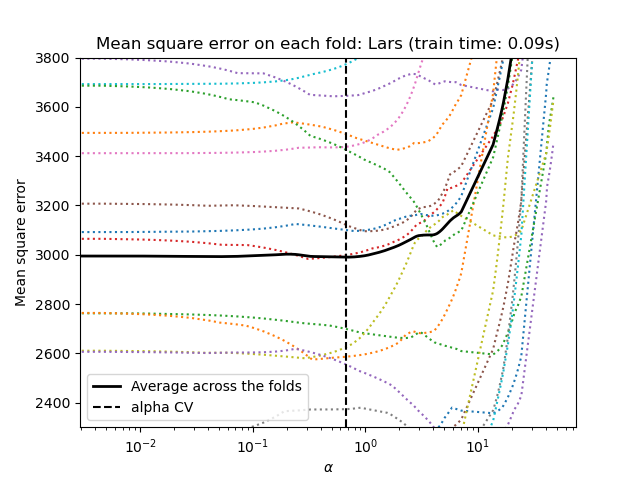# Lasso model selection: AIC-BIC / cross-validation¶

This example focuses on model selection for Lasso models that are linear models with an L1 penalty for regression problems.

Indeed, several strategies can be used to select the value of the regularization parameter: via cross-validation or using an information criterion, namely AIC or BIC.

In what follows, we will discuss in details the different strategies.

# Author: Olivier Grisel
#         Gael Varoquaux
#         Alexandre Gramfort
#         Guillaume Lemaitre


## Dataset¶

In this example, we will use the diabetes dataset.

from sklearn.datasets import load_diabetes


age sex bmi bp s1 s2 s3 s4 s5 s6
0 0.038076 0.050680 0.061696 0.021872 -0.044223 -0.034821 -0.043401 -0.002592 0.019907 -0.017646
1 -0.001882 -0.044642 -0.051474 -0.026328 -0.008449 -0.019163 0.074412 -0.039493 -0.068332 -0.092204
2 0.085299 0.050680 0.044451 -0.005670 -0.045599 -0.034194 -0.032356 -0.002592 0.002861 -0.025930
3 -0.089063 -0.044642 -0.011595 -0.036656 0.012191 0.024991 -0.036038 0.034309 0.022688 -0.009362
4 0.005383 -0.044642 -0.036385 0.021872 0.003935 0.015596 0.008142 -0.002592 -0.031988 -0.046641

In addition, we add some random features to the original data to better illustrate the feature selection performed by the Lasso model.

import numpy as np
import pandas as pd

rng = np.random.RandomState(42)
n_random_features = 14
X_random = pd.DataFrame(
rng.randn(X.shape, n_random_features),
columns=[f"random_{i:02d}" for i in range(n_random_features)],
)
X = pd.concat([X, X_random], axis=1)
# Show only a subset of the columns

age bp s3 s6 random_02 random_05 random_08 random_11
0 0.038076 0.021872 -0.043401 -0.017646 0.647689 -0.234137 -0.469474 -0.465730
1 -0.001882 -0.026328 0.074412 -0.092204 -1.012831 -1.412304 0.067528 0.110923
2 0.085299 -0.005670 -0.032356 -0.025930 -0.601707 -1.057711 0.208864 0.196861
3 -0.089063 -0.036656 -0.036038 -0.009362 -1.478522 1.057122 0.324084 0.611676
4 0.005383 0.021872 0.008142 -0.046641 0.331263 -0.185659 0.812526 1.003533

## Selecting Lasso via an information criterion¶

LassoLarsIC provides a Lasso estimator that uses the Akaike information criterion (AIC) or the Bayes information criterion (BIC) to select the optimal value of the regularization parameter alpha.

Before fitting the model, we will standardize the data with a StandardScaler. In addition, we will measure the time to fit and tune the hyperparameter alpha in order to compare with the cross-validation strategy.

We will first fit a Lasso model with the AIC criterion.

import time
from sklearn.preprocessing import StandardScaler
from sklearn.linear_model import LassoLarsIC
from sklearn.pipeline import make_pipeline

start_time = time.time()
lasso_lars_ic = make_pipeline(StandardScaler(), LassoLarsIC(criterion="aic")).fit(X, y)
fit_time = time.time() - start_time


We store the AIC metric for each value of alpha used during fit.

results = pd.DataFrame(
{
"alphas": lasso_lars_ic[-1].alphas_,
"AIC criterion": lasso_lars_ic[-1].criterion_,
}
).set_index("alphas")
alpha_aic = lasso_lars_ic[-1].alpha_


Now, we perform the same analysis using the BIC criterion.

lasso_lars_ic.set_params(lassolarsic__criterion="bic").fit(X, y)
results["BIC criterion"] = lasso_lars_ic[-1].criterion_
alpha_bic = lasso_lars_ic[-1].alpha_


We can check which value of alpha leads to the minimum AIC and BIC.

def highlight_min(x):
x_min = x.min()
return ["font-weight: bold" if v == x_min else "" for v in x]

results.style.apply(highlight_min)

AIC criterion BIC criterion
alphas
45.160030 5244.764779 5244.764779
42.300343 5208.250639 5212.341949
21.542052 4928.018900 4936.201520
15.034077 4869.678359 4881.952289
6.189631 4815.437362 4831.802601
5.329616 4810.423641 4830.880191
4.306012 4803.573491 4828.121351
4.124225 4804.126502 4832.765671
3.820705 4803.621645 4836.352124
3.750389 4805.012521 4841.834310
3.570655 4805.290075 4846.203174
3.550213 4807.075887 4852.080295
3.358295 4806.878051 4855.973770
3.259297 4807.706026 4860.893055
3.237703 4809.440409 4866.718747
2.850031 4805.989341 4867.358990
2.384338 4801.702266 4867.163224
2.296575 4802.594754 4872.147022
2.031555 4801.236720 4874.880298
1.618263 4798.484109 4876.218997
1.526599 4799.543841 4881.370039
0.586798 4794.238744 4880.156252
0.445978 4795.589715 4885.598533
0.259031 4796.966981 4891.067109
0.032179 4796.662409 4894.853846
0.019069 4794.652739 4888.752867
0.000000 4796.626286 4894.817724

Finally, we can plot the AIC and BIC values for the different alpha values. The vertical lines in the plot correspond to the alpha chosen for each criterion. The selected alpha corresponds to the minimum of the AIC or BIC criterion.

ax = results.plot()
ax.vlines(
alpha_aic,
results["AIC criterion"].min(),
results["AIC criterion"].max(),
label="alpha: AIC estimate",
linestyles="--",
color="tab:blue",
)
ax.vlines(
alpha_bic,
results["BIC criterion"].min(),
results["BIC criterion"].max(),
label="alpha: BIC estimate",
linestyle="--",
color="tab:orange",
)
ax.set_xlabel(r"$\alpha$")
ax.set_ylabel("criterion")
ax.set_xscale("log")
ax.legend()
_ = ax.set_title(
f"Information-criterion for model selection (training time {fit_time:.2f}s)"
)Model selection with an information-criterion is very fast. It relies on computing the criterion on the in-sample set provided to fit. Both criteria estimate the model generalization error based on the training set error and penalize this overly optimistic error. However, this penalty relies on a proper estimation of the degrees of freedom and the noise variance. Both are derived for large samples (asymptotic results) and assume the model is correct, i.e. that the data are actually generated by this model.

These models also tend to break when the problem is badly conditioned (more features than samples). It is then required to provide an estimate of the noise variance.

## Selecting Lasso via cross-validation¶

The Lasso estimator can be implemented with different solvers: coordinate descent and least angle regression. They differ with regards to their execution speed and sources of numerical errors.

In scikit-learn, two different estimators are available with integrated cross-validation: LassoCV and LassoLarsCV that respectively solve the problem with coordinate descent and least angle regression.

In the remainder of this section, we will present both approaches. For both algorithms, we will use a 20-fold cross-validation strategy.

### Lasso via coordinate descent¶

Let’s start by making the hyperparameter tuning using LassoCV.

from sklearn.linear_model import LassoCV

start_time = time.time()
model = make_pipeline(StandardScaler(), LassoCV(cv=20)).fit(X, y)
fit_time = time.time() - start_time

import matplotlib.pyplot as plt

ymin, ymax = 2300, 3800
lasso = model[-1]
plt.semilogx(lasso.alphas_, lasso.mse_path_, linestyle=":")
plt.plot(
lasso.alphas_,
lasso.mse_path_.mean(axis=-1),
color="black",
label="Average across the folds",
linewidth=2,
)
plt.axvline(lasso.alpha_, linestyle="--", color="black", label="alpha: CV estimate")

plt.ylim(ymin, ymax)
plt.xlabel(r"$\alpha$")
plt.ylabel("Mean square error")
plt.legend()
_ = plt.title(
f"Mean square error on each fold: coordinate descent (train time: {fit_time:.2f}s)"
)### Lasso via least angle regression¶

Let’s start by making the hyperparameter tuning using LassoLarsCV.

from sklearn.linear_model import LassoLarsCV

start_time = time.time()
model = make_pipeline(StandardScaler(), LassoLarsCV(cv=20)).fit(X, y)
fit_time = time.time() - start_time

lasso = model[-1]
plt.semilogx(lasso.cv_alphas_, lasso.mse_path_, ":")
plt.semilogx(
lasso.cv_alphas_,
lasso.mse_path_.mean(axis=-1),
color="black",
label="Average across the folds",
linewidth=2,
)
plt.axvline(lasso.alpha_, linestyle="--", color="black", label="alpha CV")

plt.ylim(ymin, ymax)
plt.xlabel(r"$\alpha$")
plt.ylabel("Mean square error")
plt.legend()
_ = plt.title(f"Mean square error on each fold: Lars (train time: {fit_time:.2f}s)")### Summary of cross-validation approach¶

Both algorithms give roughly the same results.

Lars computes a solution path only for each kink in the path. As a result, it is very efficient when there are only of few kinks, which is the case if there are few features or samples. Also, it is able to compute the full path without setting any hyperparameter. On the opposite, coordinate descent computes the path points on a pre-specified grid (here we use the default). Thus it is more efficient if the number of grid points is smaller than the number of kinks in the path. Such a strategy can be interesting if the number of features is really large and there are enough samples to be selected in each of the cross-validation fold. In terms of numerical errors, for heavily correlated variables, Lars will accumulate more errors, while the coordinate descent algorithm will only sample the path on a grid.

Note how the optimal value of alpha varies for each fold. This illustrates why nested-cross validation is a good strategy when trying to evaluate the performance of a method for which a parameter is chosen by cross-validation: this choice of parameter may not be optimal for a final evaluation on unseen test set only.

## Conclusion¶

In this tutorial, we presented two approaches for selecting the best hyperparameter alpha: one strategy finds the optimal value of alpha by only using the training set and some information criterion, and another strategy is based on cross-validation.

In this example, both approaches are working similarly. The in-sample hyperparameter selection even shows its efficacy in terms of computational performance. However, it can only be used when the number of samples is large enough compared to the number of features.

That’s why hyperparameter optimization via cross-validation is a safe strategy: it works in different settings.

Total running time of the script: ( 0 minutes 1.076 seconds)

Gallery generated by Sphinx-Gallery